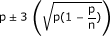1. *Transformation efficiency was estimated by the proportion (p) of transformed calli (p = x/n), where x is the number of transformed calli and n the number of co-cultivated calli. A 3 δ confidence limit for binomial distribution was calculated using the formulawith a level of confidence of 99%